# RD Sharma Solutions for Class 11 Chapter 5 - Trigonometric Functions Exercise 5.2

In Exercise 5.2 of Chapter 5, we shall discuss problems based on signs of trigonometric functions and variations in values of trigonometric functions in different quadrants. Students can refer to RD Sharma Class 11 Solutionswhich is prepared by our expert tutors to help students clarify their doubts pertaining to this chapter. On regular practice, students can speed up the method of solving problems by using short-cut tips to secure high marks in their examination. Students can download the pdf easily from the links given below.

## Download the Pdf of RD Sharma Solutions for Class 11 Maths Exercise 5.2 Chapter 5 – Trigonometric Functions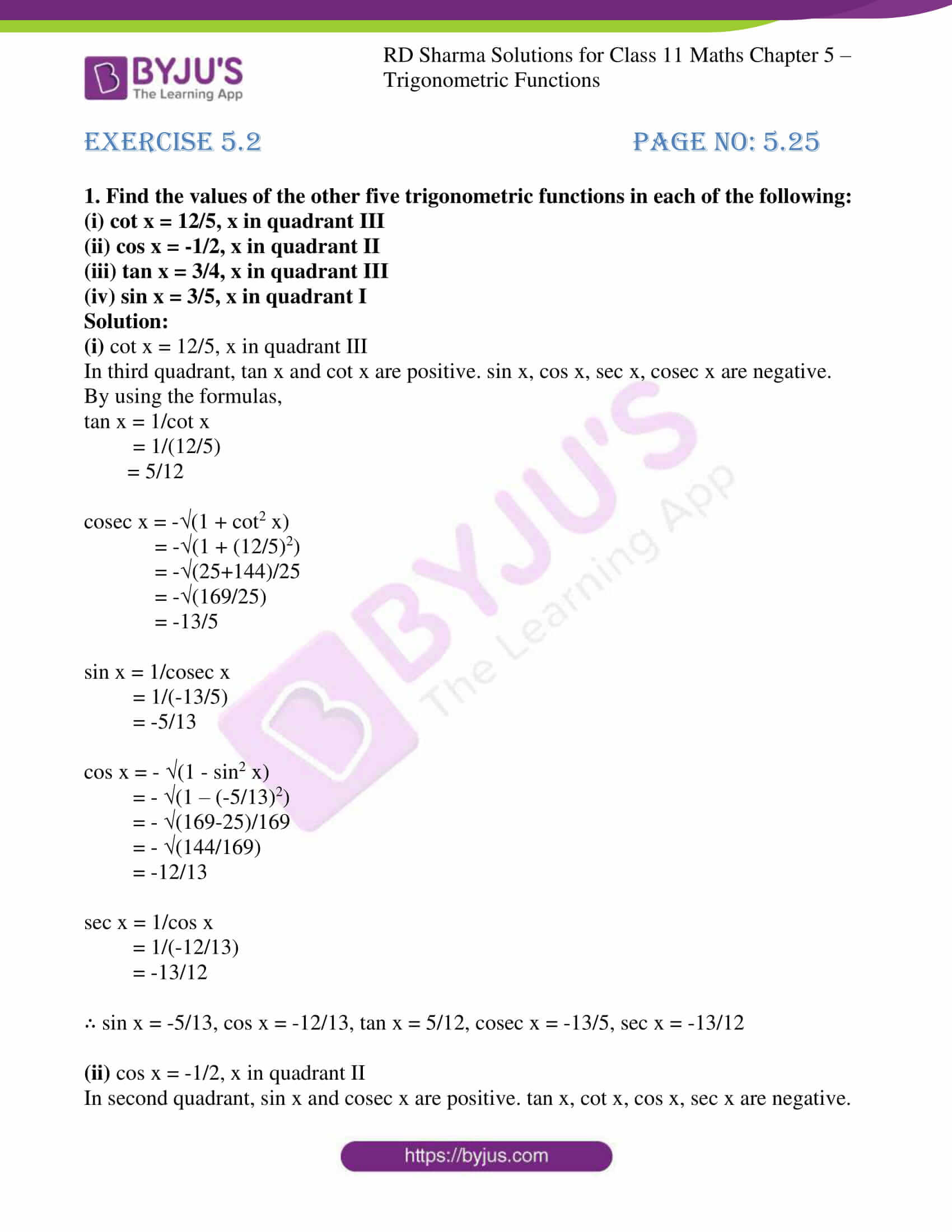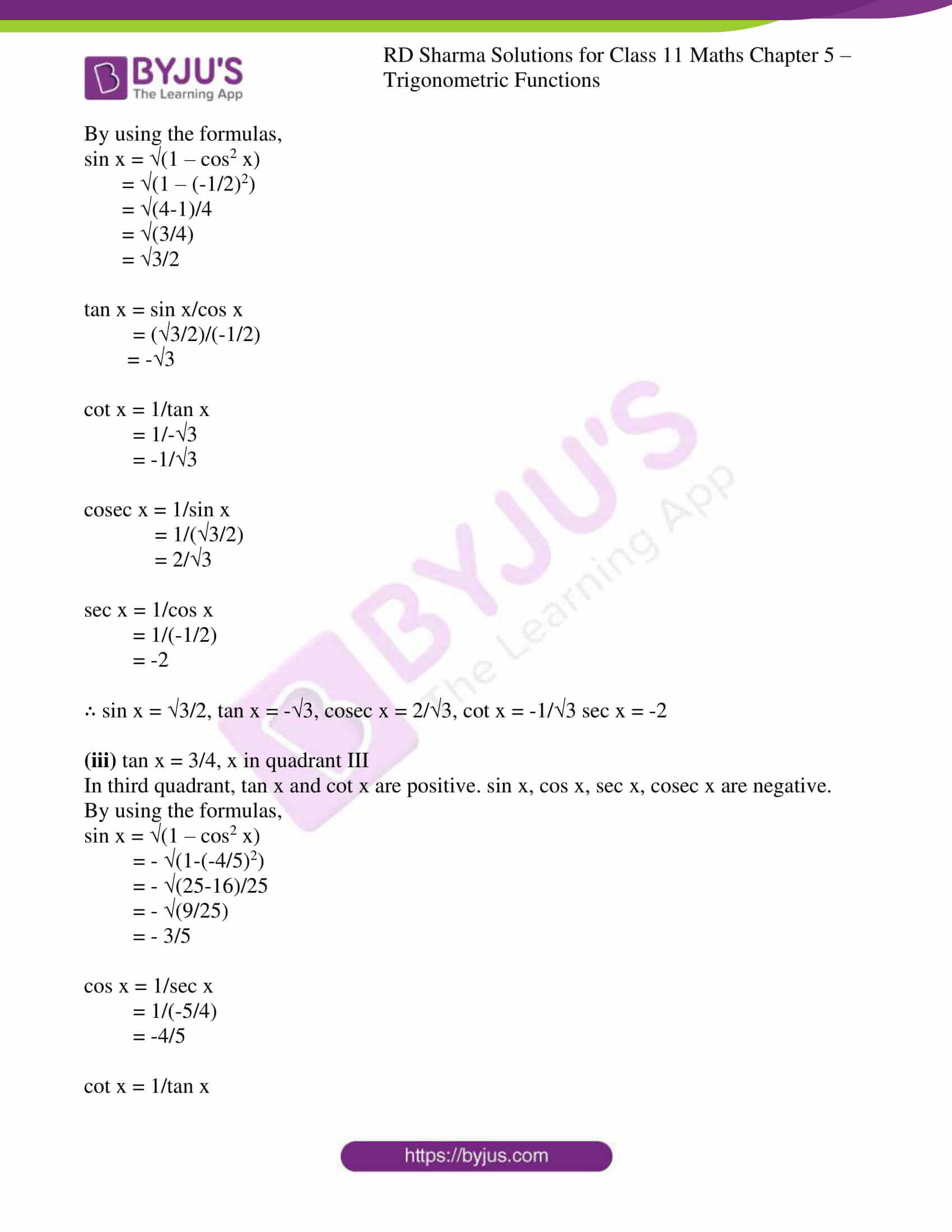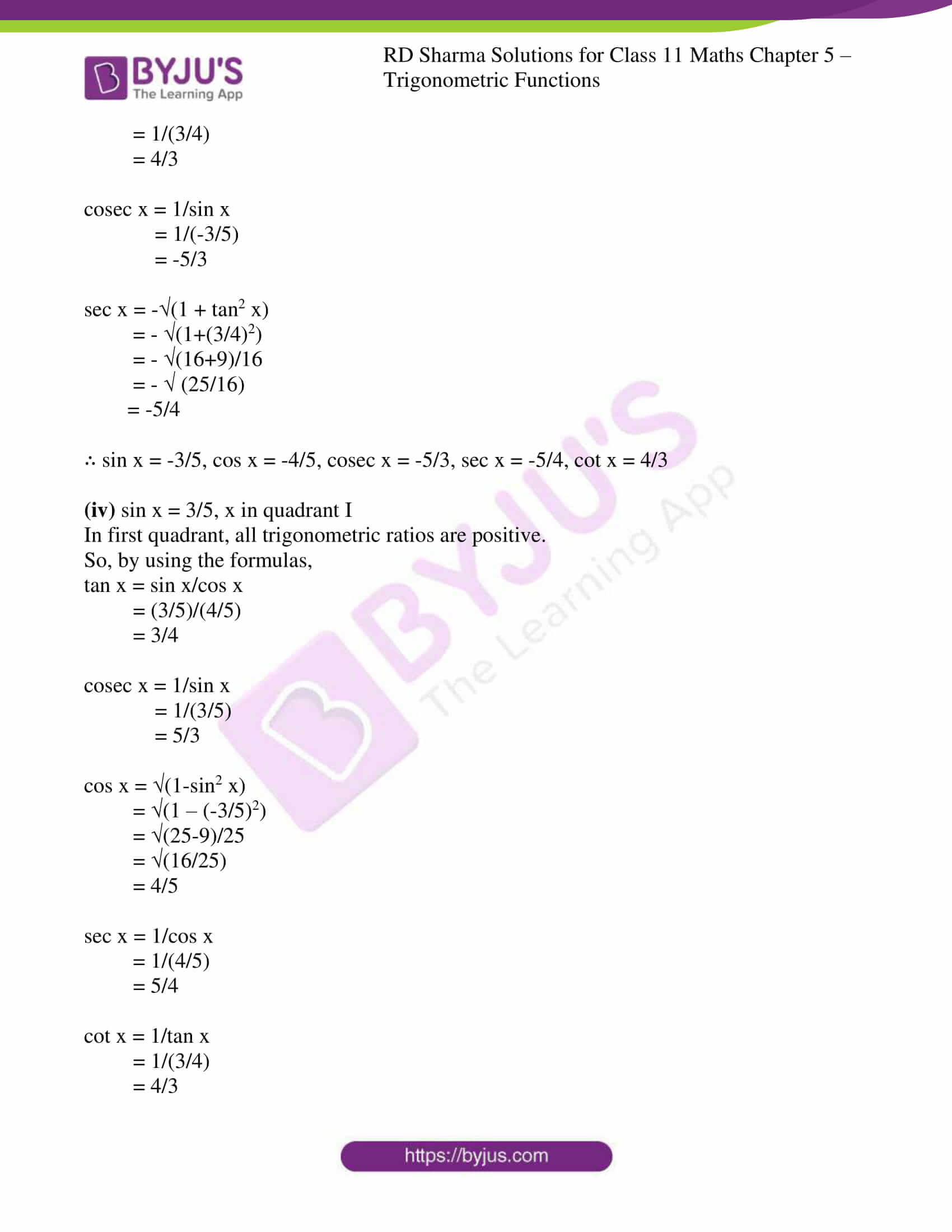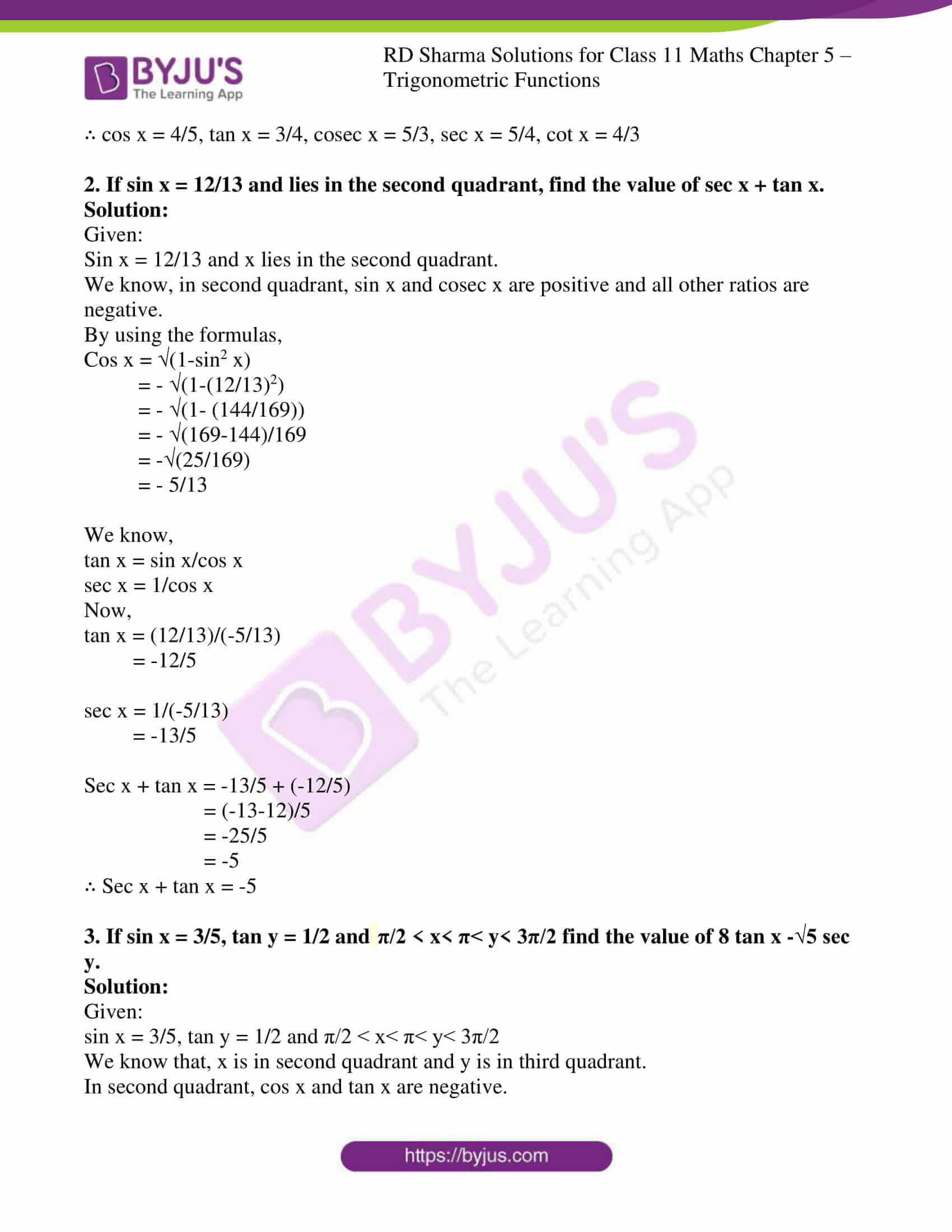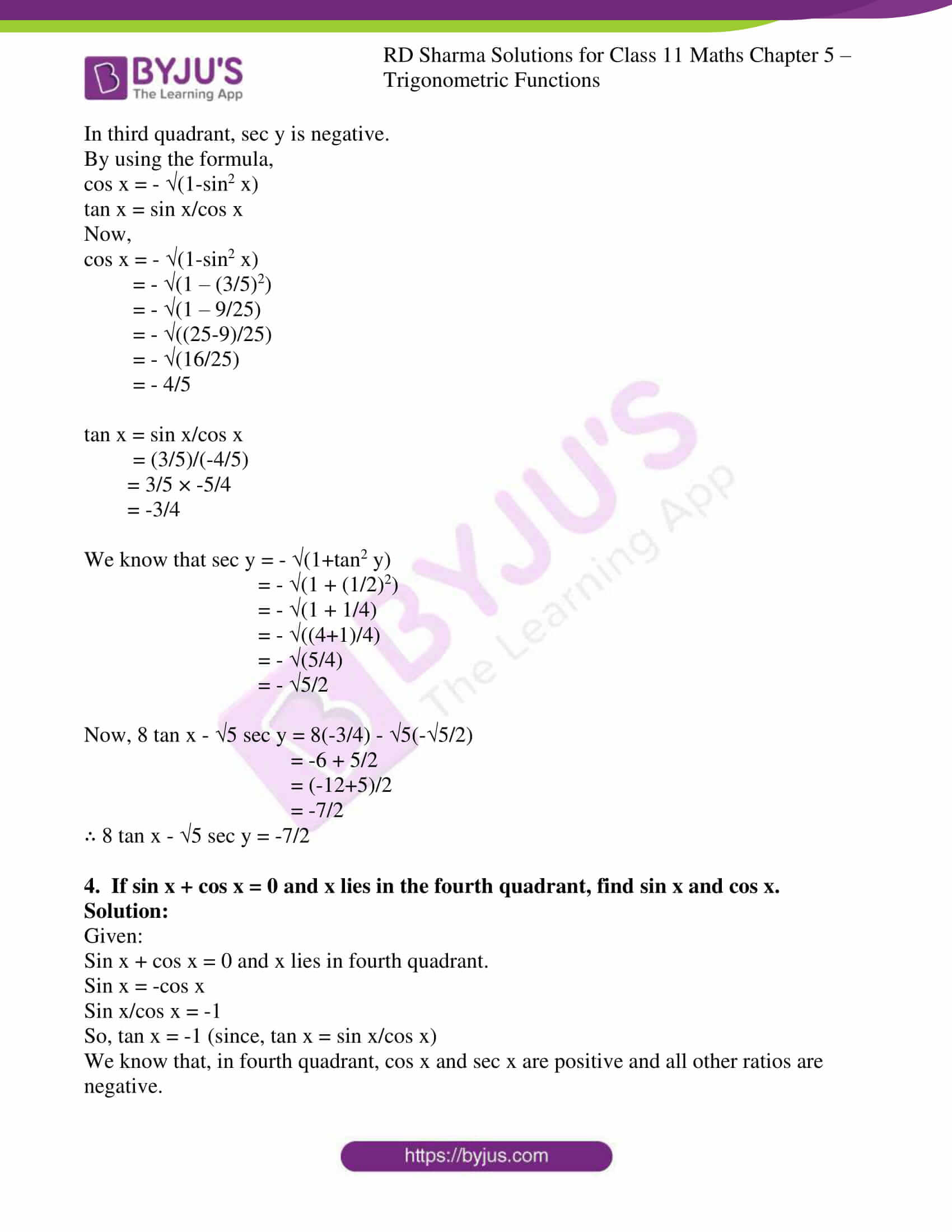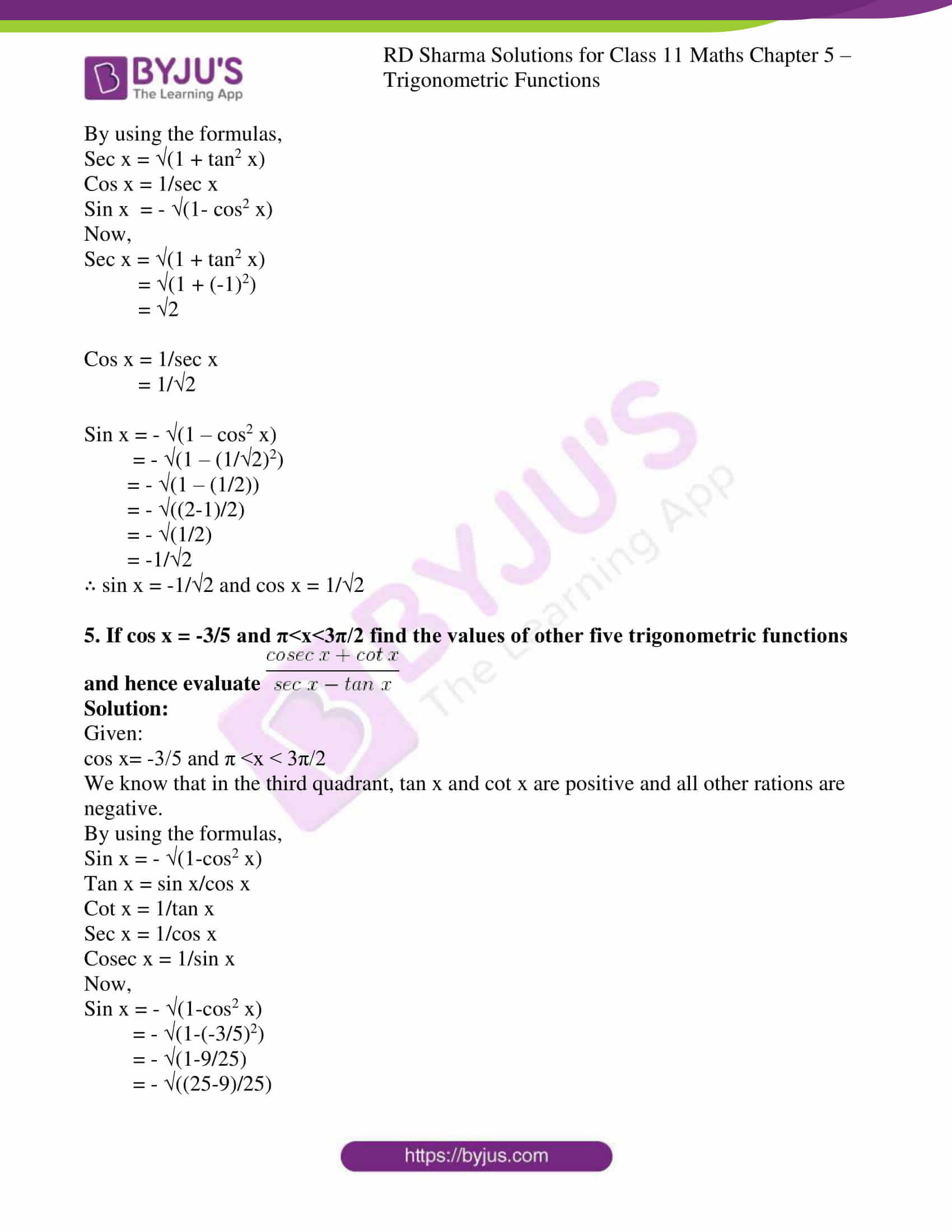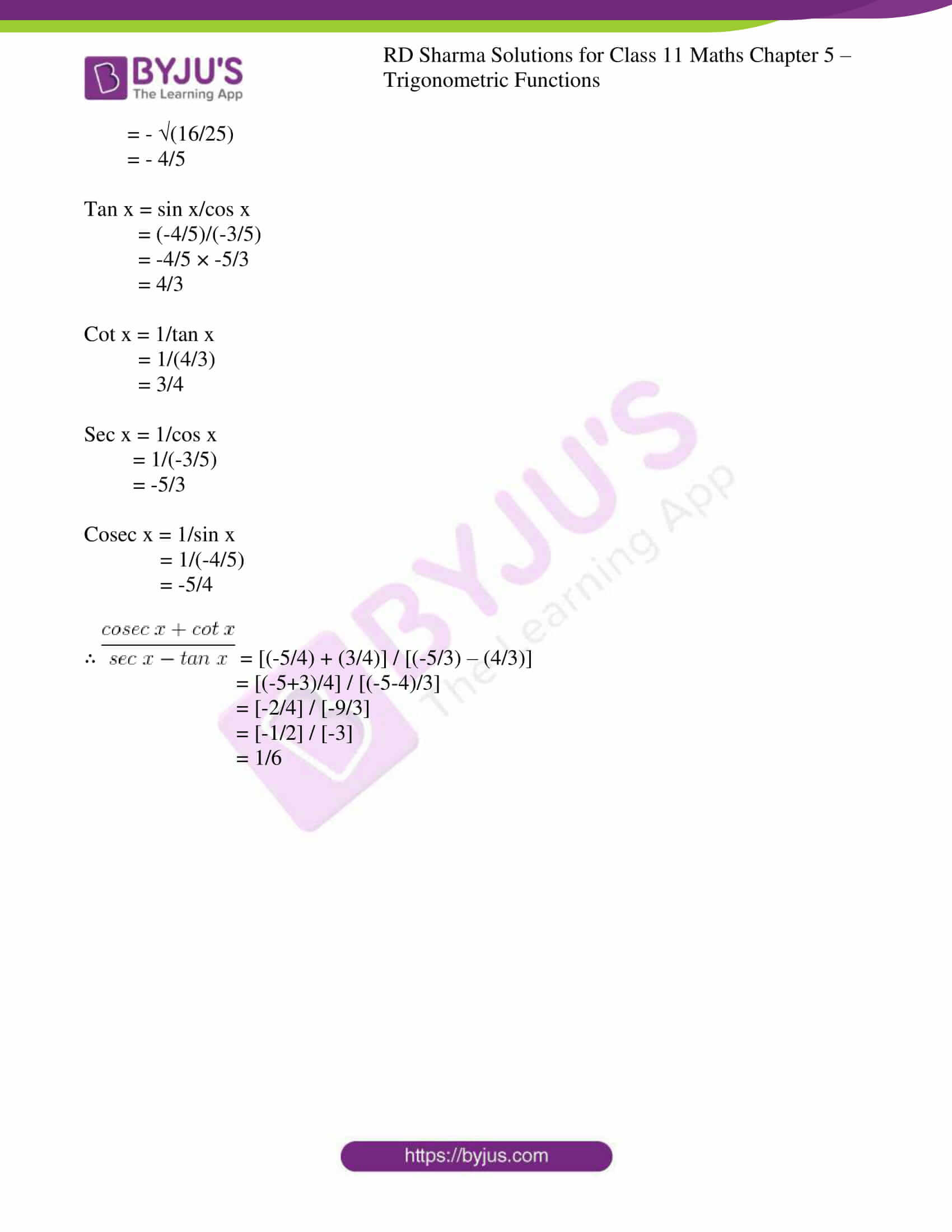### Access answers to RD Sharma Solutions for Class 11 Maths Exercise 5.2 Chapter 5 – Trigonometric Functions

1. Find the values of the other five trigonometric functions in each of the following:

(i) cot x = 12/5, x in quadrant III

(ii) cos x = -1/2, x in quadrant II

(iii) tan x = 3/4, x in quadrant III

(iv) sin x = 3/5, x in quadrant I

Solution:

(i) cot x = 12/5, x in quadrant III

In third quadrant, tan x and cot x are positive. sin x, cos x, sec x, cosec x are negative.

By using the formulas,

tan x = 1/cot x

= 1/(12/5)

= 5/12

cosec x = –(1 + cot2 x)

= –(1 + (12/5)2)

= –(25+144)/25

= –(169/25)

= -13/5

sin x = 1/cosec x

= 1/(-13/5)

= -5/13

cos x = – (1 – sin2 x)

= – (1 – (-5/13)2)

= – √(169-25)/169

= – √(144/169)

= -12/13

sec x = 1/cos x

= 1/(-12/13)

= -13/12

∴ sin x = -5/13, cos x = -12/13, tan x = 5/12, cosec x = -13/5, sec x = -13/12

(ii) cos x = -1/2, x in quadrant II

In second quadrant, sin x and cosec x are positive. tan x, cot x, cos x, sec x are negative.

By using the formulas,

sin x = √(1 – cos2 x)

= √(1 – (-1/2)2)

= √(4-1)/4

= √(3/4)

= √3/2

tan x = sin x/cos x

= (√3/2)/(-1/2)

= -√3

cot x = 1/tan x

= 1/-√3

= -1/√3

cosec x = 1/sin x

= 1/(√3/2)

= 2/√3

sec x = 1/cos x

= 1/(-1/2)

= -2

∴ sin x = √3/2, tan x = -√3, cosec x = 2/√3, cot x = -1/√3 sec x = -2

(iii) tan x = 3/4, x in quadrant III

In third quadrant, tan x and cot x are positive. sin x, cos x, sec x, cosec x are negative.

By using the formulas,

sin x = √(1 – cos2 x)

= – √(1-(-4/5)2)

= – √(25-16)/25

= – √(9/25)

= – 3/5

cos x = 1/sec x

= 1/(-5/4)

= -4/5

cot x = 1/tan x

= 1/(3/4)

= 4/3

cosec x = 1/sin x

= 1/(-3/5)

= -5/3

sec x = -√(1 + tan2 x)

= – √(1+(3/4)2)

= – √(16+9)/16

= – √ (25/16)

= -5/4

∴ sin x = -3/5, cos x = -4/5, cosec x = -5/3, sec x = -5/4, cot x = 4/3

(iv) sin x = 3/5, x in quadrant I

In first quadrant, all trigonometric ratios are positive.

So, by using the formulas,

tan x = sin x/cos x

= (3/5)/(4/5)

= 3/4

cosec x = 1/sin x

= 1/(3/5)

= 5/3

cos x = √(1-sin2 x)

= √(1 – (-3/5)2)

= √(25-9)/25

= √(16/25)

= 4/5

sec x = 1/cos x

= 1/(4/5)

= 5/4

cot x = 1/tan x

= 1/(3/4)

= 4/3

∴ cos x = 4/5, tan x = 3/4, cosec x = 5/3, sec x = 5/4, cot x = 4/3

2. If sin x = 12/13 and lies in the second quadrant, find the value of sec x + tan x.

Solution:

Given:

Sin x = 12/13 and x lies in the second quadrant.

We know, in second quadrant, sin x and cosec x are positive and all other ratios are negative.

By using the formulas,

Cos x = √(1-sin2 x)

= – √(1-(12/13)2)

= – √(1- (144/169))

= – √(169-144)/169

= -√(25/169)

= – 5/13

We know,

tan x = sin x/cos x

sec x = 1/cos x

Now,

tan x = (12/13)/(-5/13)

= -12/5

sec x = 1/(-5/13)

= -13/5

Sec x + tan x = -13/5 + (-12/5)

= (-13-12)/5

= -25/5

= -5

∴ Sec x + tan x = -5

3. If sin x = 3/5, tan y = 1/2 and π/2 < x< π< y< 3π/2 find the value of 8 tan x -√5 sec y.

Solution:

Given:

sin x = 3/5, tan y = 1/2 and π/2 < x< π< y< 3π/2

We know that, x is in second quadrant and y is in third quadrant.

In second quadrant, cos x and tan x are negative.

In third quadrant, sec y is negative.

By using the formula,

cos x = – √(1-sin2 x)

tan x = sin x/cos x

Now,

cos x = – √(1-sin2 x)

= – √(1 – (3/5)2)

= – √(1 – 9/25)

= – √((25-9)/25)

= – √(16/25)

= – 4/5

tan x = sin x/cos x

= (3/5)/(-4/5)

= 3/5 × -5/4

= -3/4

We know that sec y = – √(1+tan2 y)

= – √(1 + (1/2)2)

= – √(1 + 1/4)

= – √((4+1)/4)

= – √(5/4)

= – √5/2

Now, 8 tan x – √5 sec y = 8(-3/4) – √5(-√5/2)

= -6 + 5/2

= (-12+5)/2

= -7/2

∴ 8 tan x – √5 sec y = -7/2

4. If sin x + cos x = 0 and x lies in the fourth quadrant, find sin x and cos x.

Solution:

Given:

Sin x + cos x = 0 and x lies in fourth quadrant.

Sin x = -cos x

Sin x/cos x = -1

So, tan x = -1 (since, tan x = sin x/cos x)

We know that, in fourth quadrant, cos x and sec x are positive and all other ratios are negative.

By using the formulas,

Sec x = √(1 + tan2 x)

Cos x = 1/sec x

Sin x = – √(1- cos2 x)

Now,

Sec x = √(1 + tan2 x)

= √(1 + (-1)2)

= √2

Cos x = 1/sec x

= 1/√2

Sin x = – √(1 – cos2 x)

= – √(1 – (1/√2)2)

= – √(1 – (1/2))

= – √((2-1)/2)

= – √(1/2)

= -1/√2

∴ sin x = -1/√2 and cos x = 1/√2

5. If cos x = -3/5 and π<x<3π/2 find the values of other five trigonometric functions and hence evaluate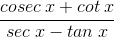Solution:

Given:

cos x= -3/5 and π <x < 3π/2

We know that in the third quadrant, tan x and cot x are positive and all other rations are negative.

By using the formulas,

Sin x = – √(1-cos2 x)

Tan x = sin x/cos x

Cot x = 1/tan x

Sec x = 1/cos x

Cosec x = 1/sin x

Now,

Sin x = – √(1-cos2 x)

= – √(1-(-3/5)2)

= – √(1-9/25)

= – √((25-9)/25)

= – √(16/25)

= – 4/5

Tan x = sin x/cos x

= (-4/5)/(-3/5)

= -4/5 × -5/3

= 4/3

Cot x = 1/tan x

= 1/(4/3)

= 3/4

Sec x = 1/cos x

= 1/(-3/5)

= -5/3

Cosec x = 1/sin x

= 1/(-4/5)

= -5/4= [(-5/4) + (3/4)] / [(-5/3) – (4/3)]

= [(-5+3)/4] / [(-5-4)/3]

= [-2/4] / [-9/3]

= [-1/2] / [-3]

= 1/6